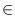Next: Node Partitions ( node_partition Up: Graphs and Related Data Previous: Sets of Edges (   Contents   Index

# Lists of Nodes ( node_list )

Definition

An instance of the data type node_list is a doubly linked list of nodes. It is implemented more efficiently than the general list type list < node > (Linear Lists). However, it can only be used with the restriction that every node is contained in at most one node_list. Also many operations supported by list < node > (for instance size) are not supported by node_list.

#include < LEDA/graph/node_list.h >

Creation

 node_list L introduces a variable L of type node_list and initializes it with the empty list.

Operations

 void L.append(node v) appends v to list L. void L.push(node v) adds v at the front of L. void L.insert(node v, node w) inserts v after w into L. Precondition wL. node L.pop() deletes the first node from L and returns it. Precondition L is not empty. node L.pop_back() deletes the last node from L and returns it. Precondition L is not empty. void L.del(node v) deletes v from L. Precondition vL. bool L.member(node v) returns true if vL and false otherwise. bool L(node v) returns true if vL and false otherwise. node L.head() returns the first node in L (nil if L is empty). node L.tail() returns the last node in L (nil if L is empty). node L.succ(node v) returns the successor of v in L. Precondition vL. node L.pred(node v) returns the predecessor of v in L. Precondition vL. node L.cyclic_succ(node v) returns the cyclic successor of v in L. Precondition vL. node L.cyclic_pred(node v) returns the cyclic predecessor of v in L. Precondition vL. bool L.empty() returns true if L is empty and false otherwise. void L.clear() makes L the empty list.

forall(x, L) { the elements of L are successively assigned to x'' }Next: Node Partitions ( node_partition Up: Graphs and Related Data Previous: Sets of Edges (   Contents   Index